Functions and CALL Routines

# BLKSHPTPRC Function

Calculates put prices for European options on stocks, based on the Black-Scholes model.
 Category: Financial

## Syntax

 BLKSHPTPRC(E, t, S, r, sigma)

### Arguments

E

is a non-missing, positive value that specifies the exercise price.

 Requirement: Specify E and S in the same units.
t

is a non-missing value that specifies the time to maturity.

S

is a non-missing, positive value that specifies the share price.

 Requirement: Specify S and E in the same units.
r

is a non-missing, positive fraction that specifies the risk-free interest rate for period t.

 Requirement: Specify a value for r for the same time period as the unit of t.
sigma

is a non-missing, positive fraction that specifies the volatility of the underlying asset.

 Requirement: Specify a value for sigma for the same time period as the unit of t.

The BLKSHPTPRC function calculates the put prices for European options on stocks, based on the Black-Scholes model. The function is based on the following relationship:
where

 S is a non-missing, positive value that specifies the share price. E is a non-missing, positive value that specifies the exercise price of the option.
where

 t specifies the time to expiration. r specifies the risk-free interest rate for period t.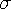specifies the volatility (the square root of the variance).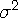specifies the variance of the rate of return.

For the special case of t=0, the following equation is true:
For information about the basics of pricing, see Using Pricing Functions.

The BLKSHPTPRC function calculates the put prices for European options on stocks, based on the Black-Scholes model. The BLKSHCLPRC function calculates the call prices for European options on stocks, based on the Black-Scholes model. These functions return a scalar value.

SAS Statements Results

`----+----1----+----2--`
```a=blkshptprc(1000, .5, 950, 4, 2);
put a;```
`16.385367922`
```b=blkshptprc(850, 1.2, 125, 3, 1);
put b;```
` 1.426971358`
```c=blkshptprc(7500, .9, 950, 3, 2);
put c;```
`273.45025684`
```d=blkshptprc(5000, -.5, 237, 3, 2);
put d;```
`           0`

 Function:Previous Page | Next Page | Top of Page